# (3) Find the volume of the solid bonded by the curves (25 marks) r=cost & y=sint,0...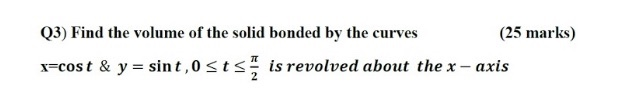(3) Find the volume of the solid bonded by the curves (25 marks) r=cost & y=sint,0 stsis revolved about the x – axis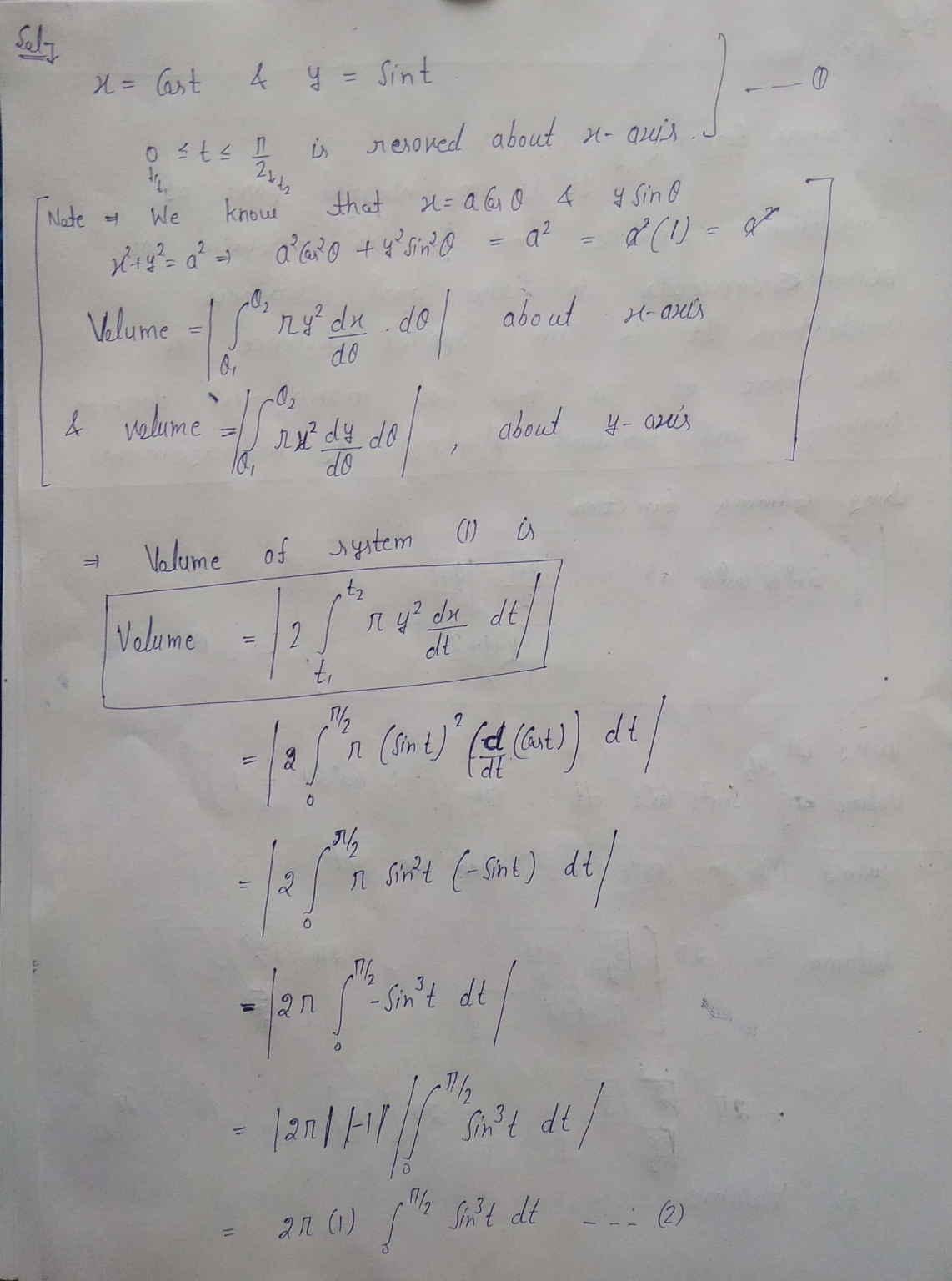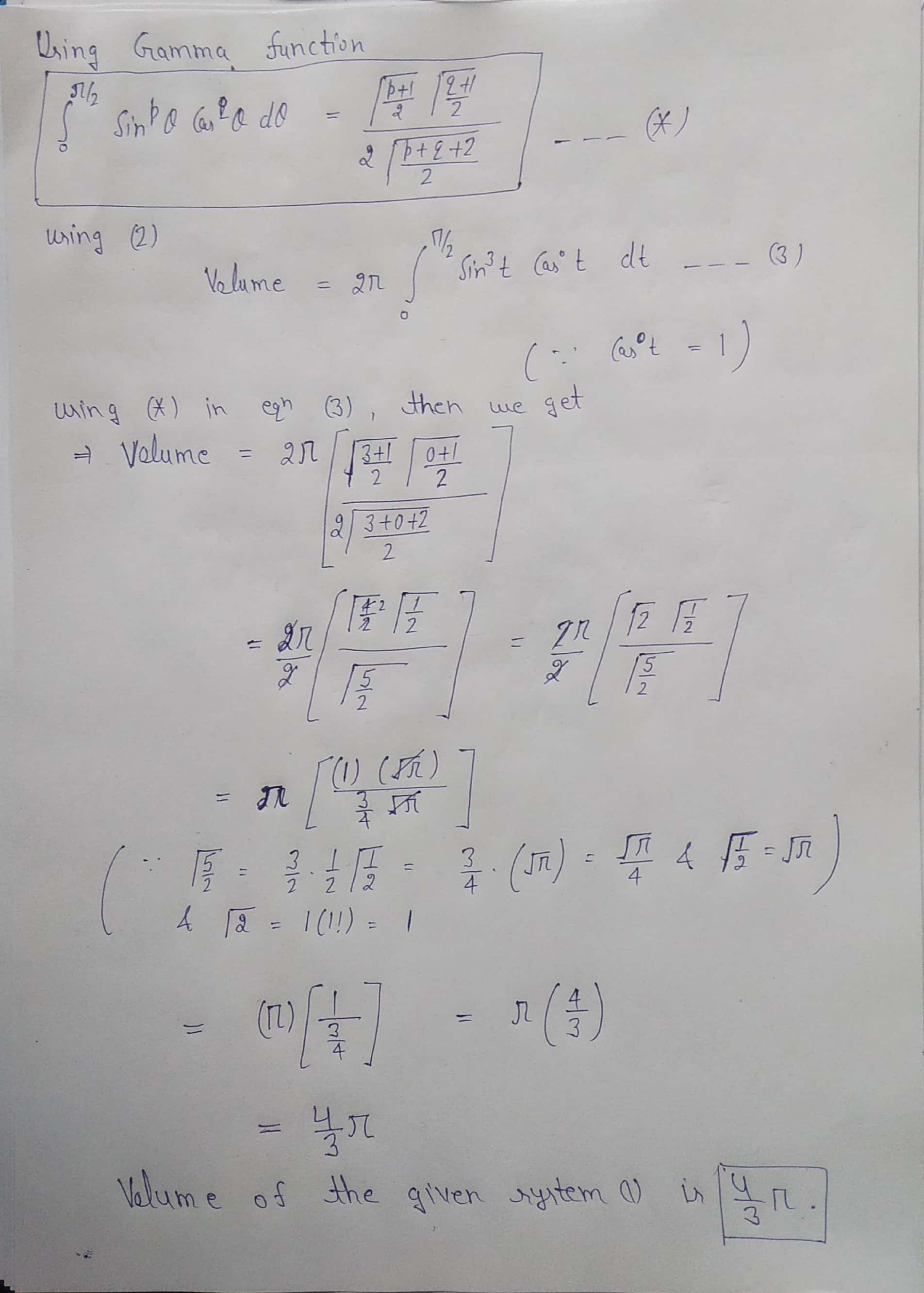##### Add Answer of: (3) Find the volume of the solid bonded by the curves (25 marks) r=cost & y=sint,0...
Similar Homework Help Questions
• ### (3) Find the volume of the solid bonded by the curves (25 marks) x=cost & y=sint,0sts...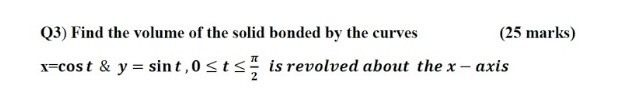(3) Find the volume of the solid bonded by the curves (25 marks) x=cost & y=sint,0sts is revolved about the x – axis

• ### Let R be the region bounded by the following curves. Use the method of your choice to find the volume of the solid gene...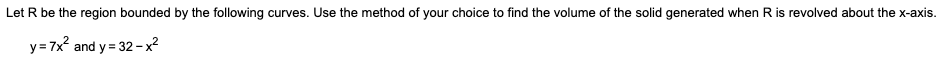Let R be the region bounded by the following curves. Use the method of your choice to find the volume of the solid generated when R is revolved about the x-axis. y=7x2 and y = 32-x2 Let R be the region bounded by the following curves. Use the shell method to find the volume of the solid generated when R is revolved about the x-axis. Let R be the region bounded by the following curves. Use the method of your...

• ### Let R be the region bounded by the following curves. Find the volume of the solid...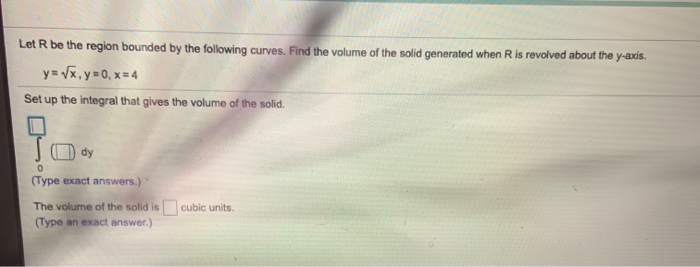Let R be the region bounded by the following curves. Find the volume of the solid generated when Ris revolved about the y-axis. y= (x,y=0, x= 4 Set up the integral that gives the volume of the solid. ody 0 (Type exact answers.) The volume of the solid is cubic units. (Type an exact answer.)

• ### Let R be the region bounded by the following curves. Find the volume of the solid generated when R is revolved about th...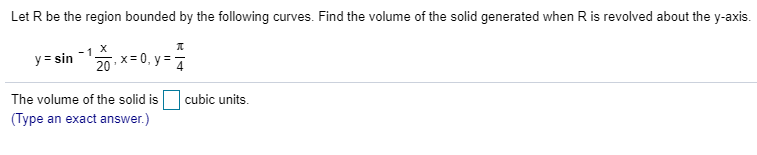Let R be the region bounded by the following curves. Find the volume of the solid generated when R is revolved about the y-axis. X -1 20 x0, y y sin cubic units The volume of the solid is (Type an exact answer.) Let R be the region bounded by the following curves. Find the volume of the solid generated when R is revolved about the y-axis. X -1 20 x0, y y sin cubic units The volume of the...

• ### Find the area of the region enclosed by the curves: x = -sec^2 y, x = sec^2 y, y = 0, y = pi/4 Using the method of cylindrical shells to find the volume of the solid that results when the region enclo...

Find the area of the region enclosed by the curves: x = -sec^2 y, x = sec^2 y, y = 0, y = pi/4 Using the method of cylindrical shells to find the volume of the solid that results when the region enclosed by the curves is revolved around the y axis. y = sqrt (x+1), y = 1, x = 1 y = 3 sqrt x, y =0, x =1 Find the volume of the solid that results when...

• ### 5. Find the volume of the solid obtained by rotating the region bounded by the curves, y = 2x, x = 0 and y = 10 abo...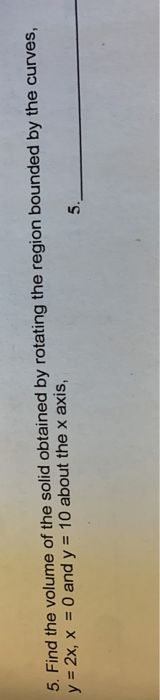5. Find the volume of the solid obtained by rotating the region bounded by the curves, y = 2x, x = 0 and y = 10 about the x axis, 5. Find the volume of the solid obtained by rotating the region bounded by the curves, y = 2x, x = 0 and y = 10 about the x axis,

• ### *********Use the method of cylindrical shells to find the volume of the solid obtained by rotating the region bounded by the curves y=x^2, y=0, x=−2 and x=−1 about the y-axis. Volume=? ***********Find...

*********Use the method of cylindrical shells to find the volume of the solid obtained by rotating the region bounded by the curves y=x^2, y=0, x=−2 and x=−1 about the y-axis. Volume=? ***********Find the volume of the solid obtained by rotating the region bounded by the given curve about the specified axis. x^2+(y−7)^2=25 about the x-axis. Volume=?

• ### Problem 2 (1) Find the area enclosed by the curves y 2 and y-4z-z2 (2) Find the volume of the solid whose base is the triangular region with vertices(0, 0), (2, 0), and (0,1). Cross-sections perpendi...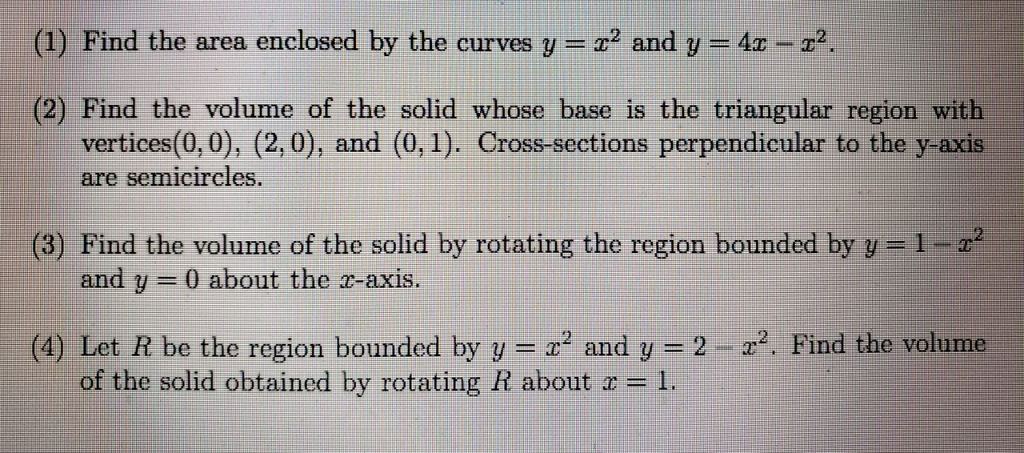Problem 2 (1) Find the area enclosed by the curves y 2 and y-4z-z2 (2) Find the volume of the solid whose base is the triangular region with vertices(0, 0), (2, 0), and (0,1). Cross-sections perpendicular to the y-axis semicircles. are (3) Find the volume of the solid by rotating the region bounded by y=1-z2 and y-0 about the r-axis. 2-z2. Find the volume (4) Let R be the region bounded by y--x2 and y of the solid obtained by...

• ### 3 1. Find the volume of the solid that results when the region enclosed by y...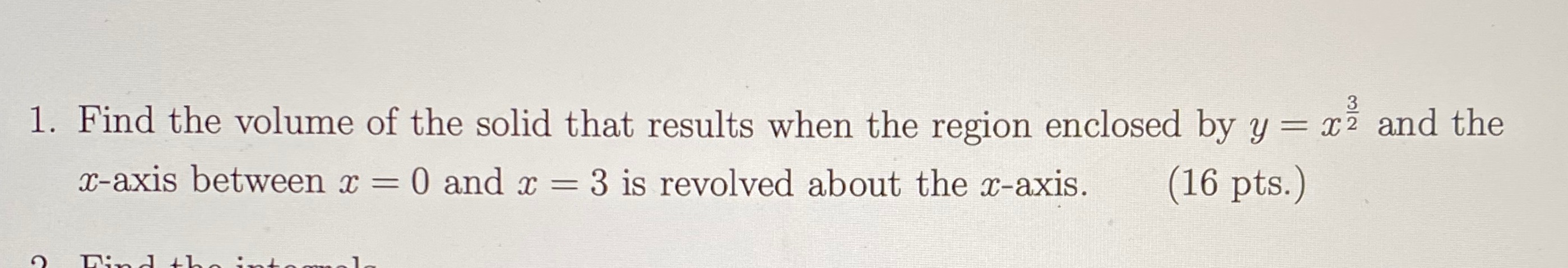3 1. Find the volume of the solid that results when the region enclosed by y = xż and the x-axis between x = 0 and x = 3 is revolved about the x-axis. (16 pts.) Dinh

• ### 4. Find the volume of the solid formed by the curves x = 1-y^4 and x= 0, and rotated about the y-axis 5. Calculate the volume of the solid obtained by rotating the region bounded by the curves y = x^2...

4. Find the volume of the solid formed by the curves x = 1-y^4 and x= 0, and rotated about the y-axis 5. Calculate the volume of the solid obtained by rotating the region bounded by the curves y = x^2, y=0, x=-2 https://gyazo.com/cedb31d3c70d20f6947f520b865a0307

Free Homework App